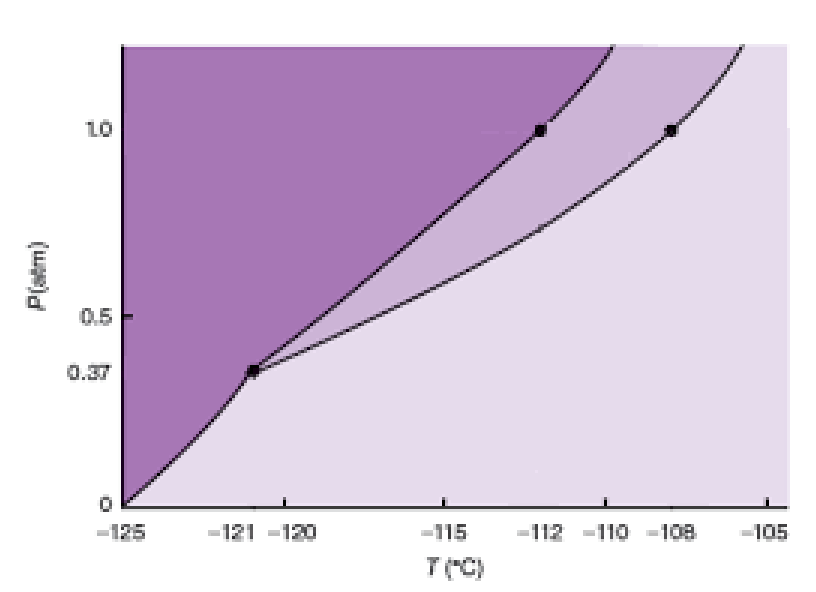# Problem: The phase diagram for xenon is shown below:In what phase is xenon found at room temperature and 1.0 atm pressure?

###### FREE Expert Solution

Analyze the given phase diagram and determine the phase of Xe at room temperature and at 1 atm

Recall that 25°C is the room temperature. Upon examining the diagram, 25°C is not part of the scaling of the diagram

89% (14 ratings)###### Problem Details

The phase diagram for xenon is shown below:In what phase is xenon found at room temperature and 1.0 atm pressure?

Frequently Asked Questions

What scientific concept do you need to know in order to solve this problem?

Our tutors have indicated that to solve this problem you will need to apply the Phase Diagram concept. You can view video lessons to learn Phase Diagram. Or if you need more Phase Diagram practice, you can also practice Phase Diagram practice problems.

What is the difficulty of this problem?

Our tutors rated the difficulty ofThe phase diagram for xenon is shown below:In what phase is ...as low difficulty.